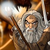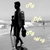+1
Beantwortet

# Using a find Index tool how can I find a character Index in different record set inputs

Anonym vor 8 Jahren in Studio / Toolbox aktualisiert von Gandalf vor 8 Jahren
When I try find index of character 2 in all record set using find Index tool
In fields as [[rec(*).a]],
Index “All Occurrence”
Character 2
And I want to put my output values in [[rec(*).b]]
Output is coming as [[rec(1).b]]=2, [[rec(2).b]]=4, [[rec(3).b]]=6, [[rec(4).b]]=8, [[rec(5).b]]=2, [[rec(6).b]]=4, [[rec(7).b]]=2,[[rec(8).b]]=4,[[rec(9).b]]=6,[[rec(10).b]]=8.
Now output is coming in record set but how would I know 2 is at index 2,4,6,8 in [[rec(1).a]] = h2f2f2f2 .
And 2 is at index 2 and 4 in [[rec(2).a]]=h2f2.
And 2 is at index 2,4,6,and 8 in [[rec(3).a]] = h2f2f2f2.

### AntwortAntwort
Beantwortet
Great example and good question.

The For Each tool is designed for this. Try dropping the Find Index tool into the for each and put the result into [[rec(*).b]].Antwort
Beantwortet
Great example and good question.

The For Each tool is designed for this. Try dropping the Find Index tool into the for each and put the result into [[rec(*).b]].Thanks I used For Each tool it works well.